/ Geometry

# Calculating Centroids of Non-Intersecting Polygons

## What Is a Centroid?

The centroid is a geometric property of a shape, somewhat related to the center of mass. It is the point denoted (x̄, ȳ) that is the average of all points in the shape. For example, in a rectangle the average of all points in the shape is dead center, as shown in Figure 1 below.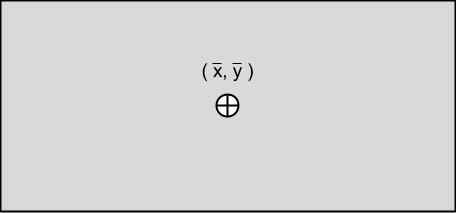Figure 1. The average position of all points, or centroid, of a rectangle is precisely in the center of the shape.

The centroid can be used as the center of mass if we assume the mass of the shape to be evenly spread throughout. With this definition, the centroid is the point at which you could balance the shape on the tip of a pencil. If the centroid is not within the shape, as in Figure 2 below, then it is not possible to balance the shape in such a way.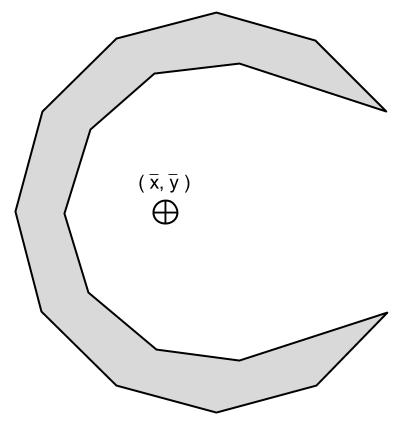Figure 2. The centroid of a crescent is not within the bounds of the shape, so there is no point on which it can be balanced.

Complex shapes can be broken down into simpler component shapes to make calculations easier. For example, we don't know intuitively how to calculate the centroid of the shape shown in Figure 3 below, but we have a formula for its rectangular pieces. The centroid of the overall shape is the weighted average of the centroids of its constituent parts. In precise terms, if we divide a shape into n regions with areas A1, A2, ..., An and centroids (x̄1, ȳ1), (x̄2, ȳ2), ..., (x̄n, ȳn), the centroid of the original shape would be: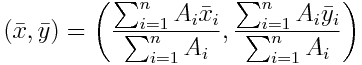Applying these equations to the T shape in Figure 3 below, we can calculate the location of the overall centroid as: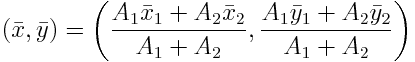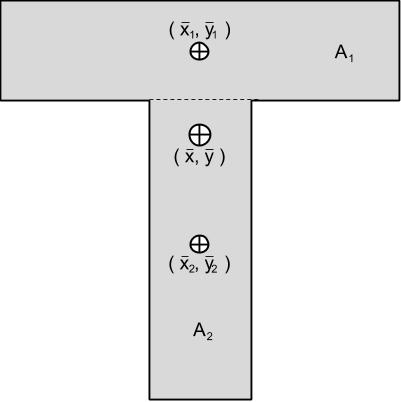Figure 3. We can find the centroid of a complex shape by taking the weighted average of the centroids of its constituent pieces.

### Why Is This Useful?

Our goal here is to find the centroid of any polygon by using a simple algorithm. This is useful because we can extend it to include shapes with curved edges, as in Figure 4 below. The centroid of a shape with smooth curved edges can be approximated by replacing the curve with a segmented approximation. We did something similar already in the crescent of Figure 2 above.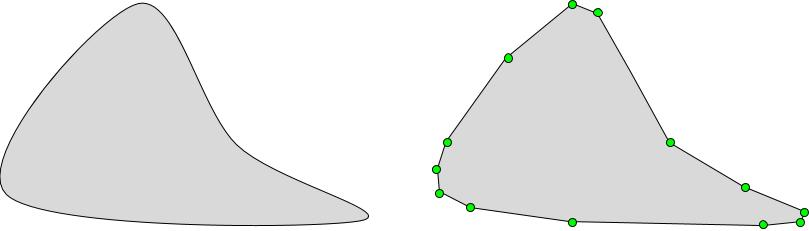Figure 4. The curved shape on the left above can be approximated by replacing its curved edges with straight line segments, forming a polygon as shown on the right. We can then approximate the centroid of the resulting polygon.

The centroid is also useful when you need to get the center of mass for an object of uniform mass distribution. This comes in handy for all sorts of things, from implementing physics in a computer game to calculating the maximum expected stress on an I-beam.

The only caveat with our algorithm is that the polygon must be non-intersecting. This is often the case, as a self-intersecting polygon doesn't model a physical object in the real world.

## What Is a Non-Intersecting Polygon?

In this context, we will define a non-intersecting polygon to be a polygon in which no two edges intersect with each other. The shapes that we typically consider such as rectangles, triangles, trapezoids, etc. are all non-intersecting. We define a self-intersecting polygon to be a shape in which at least two edges intersect with each other, such as the hourglass shape in Figure 5 below.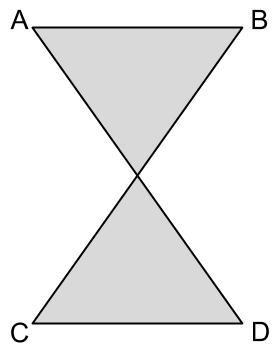Figure 5. The hourglass shape above is self-intersecting, and using it as input to our algorithm will yield incorrect results.

## The Algorithm

The polygon that we use as input to the algorithm we will denote as the ordered set of vertices P = { v1, v2, ..., vn }.

### Getting the Area and Centroid of a Triangle

The basis of our algorithm will rest upon the assumption that we know how to get the area and centroid of a triangle, given the coordinates for its three vertices. Given a triangle ABC, we can calculate the components of two vectors AB and AC, as shown in Figure 6 below.Figure 6. Going from a triangle to a pair of vectors.

By taking the cross product of the two vectors, AC × AB, we get a resulting vector that has the magnitude of the area of the parallelogram that is spanned by AB and BC. By cutting this area in half, we get just the area of the triangle ABC. This is shown in Figure 7 below.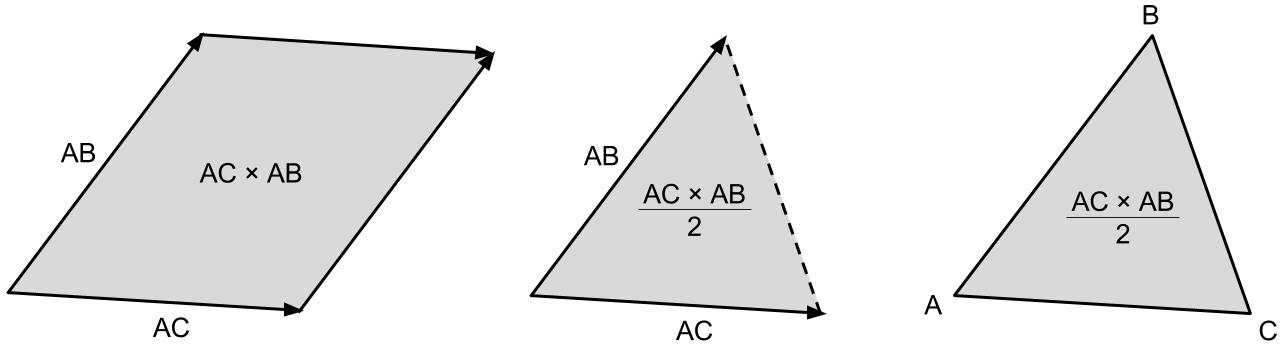Figure 7. Going from a pair of vectors back to the area of the triangle.

Because of the right-hand rule, taking the cross product AC × AB gives us a positive result if the triangle ABC is right-handed (its vertices are clockwise) and gives us a negative result if it is left-handed (its vertices are counterclockwise). However, this signed area will turn out to be necessary for our algorithm. Working out the algebra results in the following closed formula for the signed area of triangle ABC: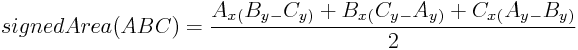Next we have to find the centroid of the triangle. We will use simple geometry to find a closed formula, although it is possible to use an algebraic argument as well. If we separate triangle ABC into n separate strips with edges parallel to side BC as shown on the left in Figure 8 below, we can use the weighted average of their centroids as the overall centroid of the triangle. As n becomes large, the strips become very thin, and their centroids approach the midpoint of their two endpoints. For the longest strip, this centroid is the midpoint of points B and C. The centroids for the other strips all follow a straight line from the midpoint of side BC through point A. Any weighted average of these points must also lie along the straight line they make.

We can make a similar argument using n strips parallel to side AB or AC to show that the centroid of the triangle must lie on the line between the midpoint of side AB and point C as well as the line between the B and the midpoint of side AC and point B. The only way for the centroid to lie on all three of these lines at once is if it is located at their intersection.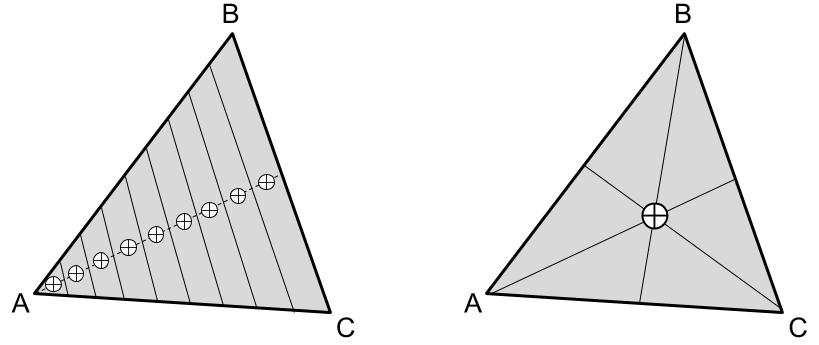Figure 8. On the left we have the triangle ABC split into strips parallel to side BC, with centroids that form a straight line from the midpoint of BC to point A. The right side shows the location of the triangle's centroid, which is at the intersection of all three such lines.

Now that we know where the centroid of a triangle is geometrically, how do we calculate it numerically from the coordinates of its vertices? Note that when we take the average of three vertices A, B, C, we can start by taking the average of any pair of the vertices to get a midpoint M. We can then average the remaining vertex with M, which must result in a point on the line between M and the remaining vertex. Since we can choose any two vertices for M, the average of A, B, C must be on the line between A and the midpoint of BC, the line between B and the midpoint of AC, and the line between C and the midpoint of AB. Therefore the average of the vertices A, B, C is precisely the centroid of the triangle ABC, as shown on the right in Figure 8 above. This gives us the following closed formula: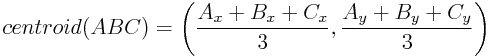### Splitting the Polygon Into Triangles

Since we have a way to calculate the area and centroid of a triangle, and we have a way to calculate the centroid of a complex shape given the areas and centroids of its component parts, the next logical step is to split the polygon P into triangles. We do this by taking any three consecutive vertices A = vi, B = vi+1, C = vi+2 in P and forming a triangle ABC. The situation so far is shown in Figure 9 below.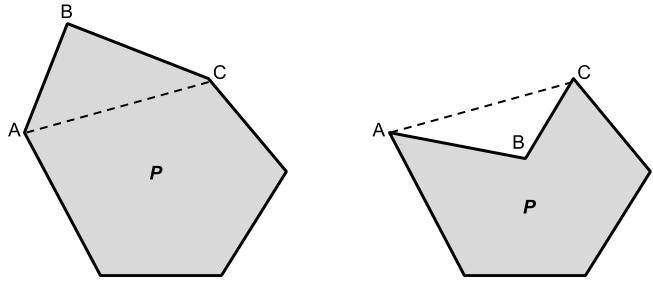Figure 9. The two possible scenarios when forming a triangle from three consecutive vertices. Either the triangle is part of the polygon P as shown on the left, or it is empty space as shown on the right.

The algorithm keeps a running count Atotal of the total area of P and a running average (x̄total, ȳtotal) of the centroid calculated so far. We add each triangle ABC's area to Atotal and average its centroid into (x̄total, ȳtotal). Next we remove the vertex B from P, forming the smaller polygon P' = P – { B }.

If the triangle ABC is part of the polygon P as on the left in Figure 9, then ABC will be right-handed and its area will be positive. Removing B removes ABC's area from ***P'***, so ABC's area and centroid will count positively toward Atotal and (x̄total, ȳtotal) overall. On the other hand, if the triangle ABC is empty space as on the right in Figure 9, then ABC will be left-handed and its area will be negative. However, removing B adds ABC's area to ***P'***, so ABC's area and centroid will count positively toward the totals due to another triangle in ***P'***. Since ABC counts negatively toward Atotal and (x̄total, ȳtotal) and then positively, it makes no impact overall. This is what we expect, as in this case ABC is empty space. The result of removing vertex B is shown in Figure 10 below.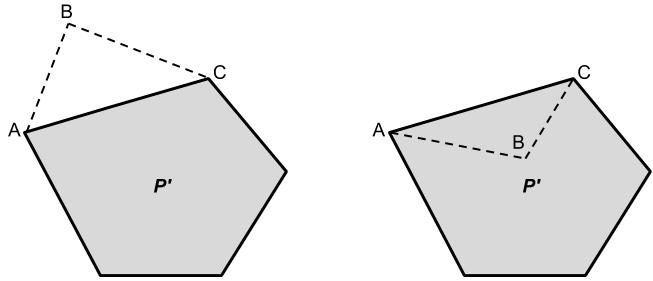Figure 10. The result of removing vertex B from the polygon P is shown above, both in the case where ABC is part of P (left) and in the case where ABC is empty space (right).

We continue in this fashion, setting P equal to ***P'***, and removing a vertex each time until P' has less than 3 vertices and thus can't form a triangle. Then Atotal and (x̄total, ȳtotal) are the overall signed area and centroid of the original polygon P.

### Runtime Analysis

The polygon begins with n vertices, and with each iteration of the algorithm it removes a vertex. Since it terminates when the polygon has less than 3 remaining vertices, it runs for a maximum of n – 2 = O(n) steps. With each step, we have to calculate the signed area and centroid of a triangle and average them into the running total for the polygon. These calculations take a constant amount of time, so overall the algorithm runs in O(n) time. This means the amount of steps the algorithm has to take increases linearly with the number of vertices in the polygon.

However, if we wanted to check the polygons to make sure that they were non-intersecting before running the algorithm, we would have to check every edge against every other edge. This would increase the run time of the algorithm to O(n2). For polygons with a large number of vertices, checking for self-intersection would dramatically increase the number of steps in the algorithm.

### Python Implementation

The following is an implementation of the algorithm in Python. Given a list of three or more points, it returns the centroid of the polygon that they form.#### Robert Fotino

I'm a former computer science student at UCLA, and a game developer in my free time. This site is a place for me to write about whatever I happen to be working on.